# Plus One Computer Science Chapter Wise Questions and Answers Chapter 6 Data Types and Operators

Students can Download Chapter 6 Data Types and Operators Questions and Answers, Plus One Computer Science Chapter Wise Questions and Answers helps you to revise the complete Kerala State Syllabus and score more marks in your examinations.

## Kerala Plus One Computer Science Chapter Wise Questions and Answers Chapter 6 Data Types and Operators

### Plus One Data Types and Operators One Mark Questions and Answers

Question 1.
___________ is the main activity carried out in computers.
Answer:
Data processingQuestion 2.
The data used in computers are different. To differentiate the nature and size of data ___________ is used.
Answer:
Data types

Question 3.
Classify the following data types,
int, array, function, char, pointer, void, float, double, structure
Answer:

 Fundamental data types Derived data types int array float function double pointer void structure char

Question 4.
Sheela wants to store her age. From the following which is the exact data type.
(a) void
(b) char
(c) int
(d) double
Answer:
(c) int

Question 5
Integer data type uses ________ bytes of memory.
(a) 5
(b) 2
(c) 3
(d) 4
Answer:
(d) 4

Question 6.
char data type ________uses bytes of memory.
(a) 1
(b) 3
(c) 7
(d) 8
Answer:
(a) 1Question 7.
From the following which data type uses 4 bytes of memory.
(a) float
(b) short
(c) char
(d) double
Answer:
(a) float

Question 8.
Full form of ASCII is ___________
Answer:
American Standard Code for Information Interchange

Question 9.
Ramu wants to store the value of From the following which is correct declaration.
(a) char pi = 3.14157
(b) int pi = 3.14157
(c) float pi = 3.14157
(d) long pi = 3.14157
Answer:
(c) float pi = 3.14157

Question 10.
From the following which is not true, to give a variable name.
(a) Starting letter must be an alphabet
(b) contains digits
(c) Cannot be a keyword
(d) special characters can be used
Answer:
(d) special characters can be used

Question 11.
Pick a valid variable name from the following.
(а) 9a
(b) float
(c) age
(d) date of birth
Answer:
(c) age.

Question 12.
To perform a unary operation how many number of operands needed?
(a) 2
(b) 3
(c) 1
(d) None of these.
Answer:
(c) 1 (Unary means one)Question 13.
To perform a binary operation how many number of operands needed?
(а) 2
(b) 3
(c) 1
(d) None of these.
Answer:
(a) 2 (binary means two)

Question 14.
To perform a ternary operation how many number of operands needed?
(a) 2
(b) 3
(c) 1
(d) None of these.
Answer:
(b) 3 (eg: ternary means three)

Question 15.
In C++ 13 % 26 =
(a) 26
(b) 13
(c) 0
(d) None of these
Answer:
(b) % is a mod operator i.e. it gives the remainder. Here the remainder is 13.

Question 16.
In C++ 41/2 =
(a) 20.5
(b) 20
(c) 1
(d) None of these
Answer:
(b) 20. (The actual result is 20.5 but both 41 and 2 are integers so .5 must be truncated)

Question 17.
++ is a __________ operator.
(a) Unary
(b) Binary
(c) Ternary
(d) None of these
Answer:
(a) Unary.

Question 18.
Conditional operator is _________ operator.
(a) Unary
(b) Binary
(c) Ternary
(d) None of these
Answer:
(c) Ternary

Question 19.
% is a _______ operator
(a) Unary
(b) Binary
(c) Ternary
(d) None of these
Answer:
(b) Binary

Question 20.
State True/False

1. Multiplication, division, modulus have equal priority
2. Logical and (&&) has less priority than logical or ()

Answer:

1. True
2. FalseQuestion 21.
_______is composed of operators and operands.
(a) expression
(b) Keywords
(c) Identifier
(d) Punctuators
Answer:
(a) expression

Question 22.
Supply value to a variable at the time of declaration is known as __________.
Answer:
Initialisation

Question 23.
From the following which is initialisation.
(a) int k;
(b) int k = 100;
(c) int k;
(d) None of these
Answer:
(b) int k = 100;

Question 24.
State True/False
In an expression all the operands having lower size are converted(promoted) to the data type of the highest sized operand.
Answer:
True

Question 25.
Classify the following as arithmetic/Logical expression.
(a) x + y * z
(b) x < y && y > z
(c) x / y
(d) x > 89 || y < 80
Answer:
(a) and (c) are Arithmetic, (b) and (d) are Logical

Question 26.
Suppose x = 5 and y = 2 then what will be cout<<(float)x/y
Answer:
2.5 The integer x is converted to float hence the result.

Question 27.
Consider the following.
a = 10;
a* =10;
Then a =
(a) a = 100
(b) a = 50
(c) a = 10
(d) a = 20
Answer:
(a) a = 100. This short hand means a = a*10

Question 28.
Consider the following.
a = 10;
a+ = 10;
Then a =
(a) a = 30
(b) a = 50
(c) a = 10
(d) a = 20
Answer:
(d) a = 20. This short hand means a = a + 10

Question 29.
Pick the odd one out
(a) structure
(b) Array
(c) Pointer
(d) int
Answer:
(d) int, it is fundamental data type the others are derived data typesQuestion 30.
From the following select not a character of C++ language
(a) A
(b) 9
(c) \
(d) @
Answer:
(d) @

Question 31.
Consider the following
float x = 25.56;
cout<<(int)x;
Here the data type of the variable is converted. What type of conversion is this?
(a) type promotion
(b) type casting
(c) implicit conversion
(d) None of these
Answer:
(b) type casting (explicit conversion);

Question 32.
Identify the error in the following C++ statement and correct it.
short population = 68000;
Answer:
The maximum number that can store in short type is less than 32767. So to store 68000 we have to use long data type.

Question 33.
Consider the following statements in C++ if(mark>=18)
cout<<“Passed”;
else
cout<<“Failed”;
Suggest an operator in C++ using which the same output can be produced.
Answer:
Conditional operator (?:)

### Plus One Data Types and Operators Two Mark Questions and Answers

Question 1.
Analyses the following statements and write True or False. Justify

1. There is an Operator in C++ having no special character in it
2. An operator cannot have more than 2 operands
3. Comma operator has the lowest precedence
4. All logical operators are binary in nature
5. It is not possible to assign the constant 5 to 10 different variables using a single C++ expression
6. In type promotion the operands with lower data type will be converted to the highest data type in expression.

Answer:

1. True (sizeof operator)
2. False(conditional operator can have 3 operands)
3. True
4. False
5. False(Multiple assignment is possible)
eg: a = b = c ==5
6. True

Question 2.
Consider the following declaration.
const int bp;
bp = 100;
Is it valid? Explain it?
Answer:
This is not valid. This is an error. A constant variable cannot be modified. That is the error and a constant variable must be initialised. So the correct declaration is as follows, const int bp = 100;

Question 3.
Consider the following statements in C++

1. cout<<41/2;
2. cout<<41/2.0;

Are this two statements give same result? Explain?
Answer:
This two statements do not give same results. The first statement 41/2 gives 20 instead of 20.5. The reason is 41 and 2 are integers. If two operands are integers the result must be integer, the real part must be truncated.

To get floating result either one of the operand must be float. So the second statement gives 20.5. The reason 41 is integer but 2.0 is a float.Question 4.
If mark = 70 then what will be the value of variable result in the following
result = mark > 50? ’P’: ’F’;
Answer:
The syntax of the conditional operator is given below
Condition ? Value if true : Value if false;
Here the conditional operator first checks the condition i.e., 70 > 50 it is true. So ’P’ is assigned to the variable result.
So the result is ’P’;

Question 5.
Is it possible to initialise a variable at the time of execution. What kind of initialisation is this? Give an example
Answer:
Yes it is possible. This is known as Dynamic initialisation. The example is given below
eg: int a = 10, b = 5; int c = a*b; here the variable c is declared and initialised with the value 10*5.

Question 6.
Boolean data type is used to store True/False in C++. Is it true? Is there any data type called Boolean in C++?
Answer:
No there is no data type for storing boolean value true/false. But in C++ non -zero (either negative or positive) is treated as true and zero is treated as false

Question 7.
Consider the following
n=-15;
if (n)
cout<<“Hello”;
else
cout<<“hai”;
What will be the output of the above code?
Answer:
The output is Hello, because n = -15 a non zero number and it is treated as true hence the result.

Question 8.
Is it possible to declare a variable in between the program as and when the need arise? Then what is it?
Answer:
Yes it is possible to declare a variable in between the program as and when the need arise. It is known as dynamic initialisation.
eg. int x = 10, y = 20;
int z = x*y;

Question 9.
char ch;
cout<<“Enter a character”;
cin>>ch;
Consider the above code, a user gives 9 to the variable ‘ch’. Is there any problem? Is it valid?
Answer:
There is no problem and it is valid since 9 is a character. Any symbol from the key board is treated as a character.

Question 10.
“With the same size we can change the sign and range of data”. Comment on this statement.
Answer:
With the help of type modifiers we can change the sign and range of data with same size. The important modifiers are signed, unsigned, long and short.

Question 11.
Write short notes about C++ short hands?
Answer:
x = x + 10 can be represented as x + = 10, It is called shorthands in C++. It is faster. This is used with all the arithmetic operators as follows.

 Arithmetic Assignment Expression Equivalent Arithmetic Expression x+ = 10 x = x + 10 x- = 10 x = x -10 X* = 10 x = x * 10 x/ = 10 x = x /10 x% = 10 x = x % 10

Question 12.
What is the role of ‘const’ modifier?
Answer:
This ‘const’ keyword is used to declare a constant.
eg: const int bp=100;
By this the variable bp is treated as constant and cannot be possible to change its value during execution.Question 13.
Specify the most appropriate data type for handling the following data.

1. Roll no. of a student.
2. Name of an employee.
3. Price of an article.
4. Marks of 12 subjects

Answer:

1. short Roll no;
2. char name;
3. float price;
4. short marks;

Question 14.
Write C++ statement for the following,

1. The result obtained when 5 is divided by 2.
2. The remainder obtained when 5 is divided by 2.

Answer:

1. 5/2
2. 5 % 2

Question 15.
Predict the output of the following code. Justify.
int k = 5;
b = 0;
b = k++ + ++k;
cout<<b;
Answer:
Output is 12. In this statement first it take the value of k in 5 then increment it K++. So first operand for + is 5. Then it becomes 6. Then ++k makes it 7. This is the second operand. Hence the result is 12.

Question 16.
Predict the output.
1. int sum = 10, ctr = 5;
sum = sum + ctr–;
cout<<sum;

2. int sum = 10, ctr = 5;
sum = sum + ++ctr;
cout<<sum;
Answer:

1. 15
2. 16

Question 17.
Predict the output.
int a;
float b;
a = 5;
cout<<sizeof(a + b/2);
Answer:
Output is 4. Result will be the memory size of floating point numberQuestion 18.
Predict the output.
int a, b, c;
a = 5;
b = 2;
c = a/b;
cout<<c;
Answer:
Output is 2. Both operands are integers. So the result will be an integer

Question 19.
Explain cascading of i/o operations
Answer:
The multiple use of input or output operators in a single statement is called cascading of i/o operators.
eg: To take three numbers by using one statement is as follows
cin>>x>y>>z;
To print three numbers by using one statement is as follows
cout<<x<<y<<z;

Question 20.
Trace out and correct the errors in the following code fragments

1. cout<<“Mark =”45;
2. cin<<“Hellow World!”;
3. cout>>”X + Y;
4. Cout<<‘Good,<<‘Morning’

Answer:

1. cout<<“Mark = 45”;
2. cout<<“Hellow World!”;
3. cout<<X + Y;
4. Cout<<“Good Morning”;

Question 21.
Raju wants to add value 1 to the variable ‘p’ and store the new value in ‘p’ itself. Write four different statements in C++ to do the task.
Answer:

1. P=P+1;
2. p++;(post increment)
3. ++p;(pre increment)
4. p+=1;(short hand in C++)

Question 22.
Read the following code
char str ;
cin>>str;
cout<<str;
If we give the input “Green Computing”, we get the output “Green”. Why is it so? How can you correct that? (2)
Answer:
The input statement cin>> cannot read the space. It reads the text up to the space, i.e. the delimiter is space. To read the text up to the enter key gets() or getline() is used.

Question 23.

 Name Symbol (a) Modulus operator (i) ++ (b) Logical Operator (ii) == (c) Relational Operator (iii) = (d) Assignment operator (iv) ?: (e) Increment operator (v) && (f) Conditional Operator (vi) %

Answer:

 Name Symbol (a) Modulus operator (vi) % (b) Logical Operator (v) && (c) Relational Operator (ii) == (d) Assignment operator (iii) = (e) Increment operator (i) ++ (f) Conditional Operator (iv) ?:Question 24.
Write a C++ expression to. calculate the value of the following equation.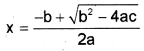Answer:
x = (-b + sqrt(b*b – 4*a*c)/(2*a)

Question 25.
A student wants to insert his name and school address in the C++ program that he has written. But this should not affect the compilation or execution of the program. How is it possible? Give an example.
Answer:
He can use comments to write this information. In C++ comments are used to write information such as programmer’s name, address, objective of the codes etc. in between the actual codes. This is not the part of the programme. There are two types of comments

1. Single line (//) and
2. Multi-line (/* and *f)

1. Single line (if):
Comment is used to make a single line as a comment. It starts with //.
eg: /./programme starts here.

2. Multi-line (/* and */):
To make multiple lines as a comment. It starts with /* and ends with */.
Eg: /* this programme is used to find sum of two numbers */

Question 26.
Consider the following C++ statements:
char word ;
cin>>word;
cout<<word;
gets(word);
puts(word);
If the string entered is “HAPPY NEW YEAR”, predict the output and jsutify your answer.
Answer:
cin>>word;
cout<<word;
It displays “HAPPY” because cin takes characters upto the space. That is space is the delimiter for cin. The string after space is truncated. To resolve this use gets () function. Because gets () function reads character upto the enter key.
Hence
gets(word);
puts(word);
Displays “HAPPY NEW YEAR”Question 27.
Write the difference between x = 5 and x == 5 in C++.
Answer:
x = 5 means the value 5 of the RHS is assigned to the LHS variable x . Here = is the assignment operator. But x == 5, == this is the relational (comparison) operator. Here it checks whether the value of RHS is equal to the value of LHS and this expression returns a boolean value as a result. It is the equality operation.

Question 28.
1. What is the output of the following program?
# include <iostream.h>
void main ()
{
int a;
a = 5 + 3*5;
cout << a;
}

2. How do 9, ‘9’ and “9” differ in C++ program?
Answer:
Here multiplication operation has more priority than addition.
hence
1. a = 5 + 15 = 20

2. Here 9 is an interger
‘9’ is a character
“9” is a string

Question 29.
Read the following C++ program and predict the output by explaining the operations performed.
#include<iostream.h>
void main ()
{
int a = 5, b = 3;
cout<<a++ /–b;
cout<<a/ (float) b;
}
Answer:
Here a = 5 and b = 3
a++ /– b = 5/2 = 2
That is a++ uses the value 5 and next it changes its value to 6
So a/(float) b = 6/(float)2
= 6/2.0
= 3
So the output is 2 and 3

Question 30.
What is the preprocessor directive statement? Explain with an example.
Answer:
A C++ program starts with the preprocessor directive i.e., # include, #define, #undef, etc, are such a preprocessor directives. By using #include we can link the header files that are needed to use the functions. By using #define we can define some constants.
eg. #define x 100. Here the value of x becomes 100 and cannot be changed in the program. No semicolon is needed.Question 31.
The following C++ code segment is a part of a program written by Smitha to find the average of 3 numbers.
int a, b, c;
float avg;
cin>>a>>b>>c;
avg = (a + b + c)/3;
cout<<avg; .
What will be the output if she inputs 1, 4 and 5? How can you correct it?
Answer:
= (1 + 4 + 5)/3
= 10/3
= 3.3333
Instead of this 3.3333 the output will be 3. This is because if both operands are integers an integer division will be occurred, that is the fractional part will be truncated. To get the correct output do as follows
case 1: int a,b,c; is replaced by float a,b,c;

OR

case 2: Replace (a + b + c)/3 by (a + b + c)/3.0;

OR

case 3: Type casting.
Replace avg = (a + b + c)/3;
by avg = (float)(a + b + c)/3;

### Plus One Data Types and Operators Three Mark Questions and Answers

Question 1.
In a panchayath or municipality all the houses have a house number, house name and members. Similar situation is in the case of memory. Explain
Answer:
The named memory locations are called variable. A variable has three important things

1. variable name: A variable should have a name
2. Memory address: Each and every byte of memory has an address. It is also called location (L) value
3. Content: The value stored in a variable is called content.lt is also called Read(R) value.

Question 2.
Briefly explain constants.
Answer:
A constant or a literal is a data item its value doe not change during execution. The keyword const is used to declare a constant. Its declaration is as follows
const data type variable name = value;
eg.const int bp = 100;
\const float pi = 3.14157;
const char ch = ‘a’;
const char[]=”Alvis”;

1. Integer literals:
Whole numbers without fractional parts are known as integer literals, its value does not change during execution. There are 3 types decimal, octal and hexadecimal.
eg: For decimal 100, 150, etc.
For octal 0100, 0240, etc.
For hexadecimal 0x100, 0x1A, etc.

2. Float literals:
A number with fractional parts and its value does not change during execution is called floating point literals.
eg: 3.14157, 79.78, etc

3. Character literal:
A valid C++ character enclosed in single quotes, its value does not change during execution.
eg: ‘m’, ‘f, etc.

4. String literal:
One or more characters enclosed in double quotes is called string constant. A string is automatically appended by a null character(‘\0’)
eg: “Mary’s”, “India”, etc.Question 3.
Consider the following statements
int a = 10, x = 20;
float b = 45000.34, y = 56.78;
1. a = b;
2. y = x;
Is there any problem for the above statements? What do you mean by type compatibility?
Answer:
Assignment operator is used to assign the value of RHS to LHS. Following are the two chances
(a) The size of RHS is less than LHS. So there is no problem and RHS data type is promoted to LHS. Here it is compatible.

(b) The size of RHS is higher than LHS. Here comes the problem sometimes LHS cannot possible to assign RHS. There may be a chance of wrong answer. Here it is not compatible.
Here
1. a = b; There is an error since the size of LHS is 2 but the size of RHS is 4.
2. y = x; There is no problem because the size of LHS is 4 and RHS is 2.

Question 4.
A company has decided to give incentives to their salesman as perthe sales. The criteria is given below.
If the total sales exceeds 10,000 the incentive is 10%

1. If the total sales >= 5,000 and total sales <10,000, the incentive is 6 %
2. If the total sales >= 1,000 and total sales <5,000, the incentive is 3 %

Write a C++ program to solve the above problem and print the incentive after accepting the total sales of a salesman. The program code should not make use of ‘if’ statement.
Answer:
#include<iostream>
using namespace std;
int mainO
{
float sales,incentive;
cout<<“enter the sales”;
cin>>sales;
incentive = (sales>10000 ? sales*.10: (sales > =5000 ? sales * .06 : (sales >= 1000 ? sales * -03: 0)));
cout<<“\nThe incentive is ” << incentive;
}

Question 5.
A C++ program code is given below to find the value of X using the expression
$$x=\frac{a^{2}+b^{2}}{2 a}$$
where a and b are variables

#include<iostream>
using namespace std;
int main()
{
int a;b;
float x
cout<<“Enter the values of a and b;
cin>a>b;
x = a*a + b*b/2*a;
cout>>x;
}
Predict the type of errors during compilation, execution and verification of the output. Also write the output of two sets of input values

1. a = 4, b = 8
2. a = 0, b = 2

Answer:
This program contains some errors and the correct program is as follows.
#include<iostream>
using namespace std;
int main()
{
int a,b;
float x; .
cout<<“Enterthe values of a and b”;
cin>>ab;
x=(a*a + b*b)/(2*a);
cout<<x;
}
The output is as follows

1. a = 4 and b = 8 then the output is 10
2. a = 0 and b = 2 then the output is an error divide by zero error(run time error)Question 6.
A list of data items are given below
45, 8.432, M, 0.124,8 , 0, 8.1 × 1031, 1010, a, 0.00025, 9.2 × 10120, 0471,-846, 342.123E03

1. Categorise the given data under proper headings of fundamental data types in C++.
2. Explain the specific features of each data type. Also mention any other fundamental data type for which sample

data is not given
Answer:
1.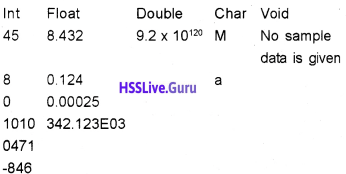2. The specific features of each data type.
(i) int data type:
It is used to store whole numbers without fractional (decimal point) part. It can be either negative or positive. It con¬sumes 4 bytes (32 bits) of memory.i.e. 232 . numbers. That is 231 negative numbers and 231 positive numbers (0 is considered as +ve ) So a total of 232 numbers. We can store a number in between -231 to + 231-1.

(ii) char data type:
Any symbol from the keyboard, eg. ‘A’ , ‘?’, ‘9’,…. It consumes one byte( 8 bits) of memory. It is internally treated as integers, i.e. 28 = 256 characters. Each character is having a ASCII code, ‘a’ is having ASCII code 97 and zero is having ASCII code 48.

3. float data type:
It is used to store real numbers i.e. the numbers with decimal point. It uses 4 bytes(32 bits) of memory.
eg: 67.89, 89.9 E-15.

4. double data type:
It is used to store very large real numbers. It uses 8 bytes(64 bits) of memory.

5. void data type:
void means nothing. It is used to represent a function returns nothing.

Question 7.
Write valid reasons after reading the following statements in C++ and comment on their correctness by give reasons.

1. char num = 66;
char num = B’;
2. 35 and 35L are different
3. The number 14, 016 and OxE are one and the same
4. Char data type is often said to be an integer type
5. To store the value 4.15 float data type is preferred over double

Answer:

1. The ASCII number of B is 66. So it is equivalent.
2. 35 is of integer type but 35L is Long
3. The decimal number 14 is represented in octal is 016 and in hexadecimal is OXE.
4. Internally char data type stores ASCII numbers.
5. To store the value 4.15 float data type is better because float requires only 4 bytes while double needs 8 bytes hence we can save the memory.Question 8.
Suggest most suitable derived data types in C++ for storing the following data items or statements

1. Age of 50 students in a class
2. Address of a memory variable
3. A set of instructions to find out the factorial of a number
4. An alternate name of a previously defined variable.
5. Price of 100 products in a consumer store
6. Name of a student

Answer:

1. Integer array of size 50
2. Pointer variable
3. Function
4. Reference
5. Float array of size 100
6. Character array

Question 9.
Considering the following C++ statements. Fill up the blanks

1. If p = 5 and q = 3 then q%p is _______
2. If E1 is true and E2 is False then E1 && E2 will be _______
3. If k = 8, ++k < = 8 will be ________
4. If x = 2 then (10* ++x) % 7 will be ________
5. If t = 8 and m = (n=3,t-n), the value of m will be ______
6. If i = 12 the value i after execution of the expres¬sion i+ = i- – + – -i will be ______

Answer:

1. 3
2. False
3. False(++k makes k = 9. So 9<=8 is false)
4. 2(++x becomes 3 ,so 10 * 3 = 30%7 = 2)
5. 5( here m = (n = 3,8-3) = (n = 3,5), so m = 5, The maximum value will take)
6. Here i = 12

i + = i- – + – -i
here post decrement has more priority than pre decrement. So “i- -” will be evaluated first. Here first uses the value then change so it uses the value 12 and i becomes 11
i + = 12 + – -i
now i = 11.
Here the value of i will be changed and used so “i- -” becomes 10
i + = 12 + 10 = 22
So, i = 22 +10
i = 32
So the result is 32.

Question 10.
The Maths teacher gives the following problem to Riya and Raju.
x = 5 + 3 * 6.
Riya got x = 48 and Raju got x = 23. Who is right and why it is happened? Write down the operator precedence in detail?
Answer:
Here the answer is x = 23. It is because of precedence of operators. The order of precedence of operators are given below.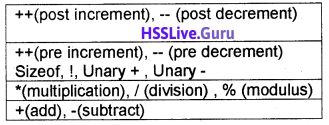Here multiplication has more priority than additionQuestion 11.
Explain the data types’ in C++. (3)
Answer:
Fundamental data types:
It is also called built-in data type. They are int, char, float, double and void
1. int data type:
It is used to store whole numbers without fractional (decimal point) part. It can be either negative or positive. It consumes 4 bytes (32 bits) of memory. i.e. 232 numbers. That is 231 negative numbers and 231 positive numbers (0 is considered as +ve) So a total of 232 numbers. We can store a number in between -231 to + 2311.

2. char data type Any symbol from the keyboard, eg. ‘A’,‘9’, …. It consumes one byte( 8 bits) of memory. It is internally treated as integers, i.e. 28 = 256 characters. Each character is having an ASCII code, ‘a’ is having ASCII code 97 and zero is having ASCII code 48.

3. float data type:
It is used to store real numbers i.e. the numbers with decimal point. It uses 4 bytes(32 bits) of memory.
eg: 67.89, 89.9 E-15.

4. double data type:
It is used to store very large real numbers. It uses 8 bytes(64 bits) of memory.

5. void data type:
Void means nothing. It is used to represent a function returns nothing.

• User defined Data types: C++ allows programmers to define their own data type. They are Structure(struct), enumeration (enum), union, class, etc.
• Derived data types: The data types derived from fundamental data types are called Derived data types. They are Arrays, pointers, functions, etc

Question 12.
Predict the output of the following C++ statements.
int a = -5, b = 3, c = 4;
C+ = a++ + –b;
cout<<a<<b<<c;
Answer:
a = -4, b = 2 and c = 1.

Question 13.
Match the followingAnswer: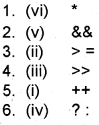Question 14.
Write any five unary operators of C++. Why are they called so?
Answer:
A unary operator is an operator that need only one operand to perform the operation. The five unary operators of C++ are given below.
Unary +, Unary -, ++, – – and ! (not)Question 15.
Write C++ examples for the following:

1. Declaration statement
2. Assignment statement
3. Type casting

Answer:

1. int age;
2. age = 16;
3. avg = (float)a + b + c/3;

### Plus One Data Types and Operators Five Mark Questions and Answers

Question 1.

• Name: Jose
• Roil no: 20
• Age: 17
• Weight: 45.650

Consider the above data, we know that there are different types of data are used in the computer. Explain different data types used in C++.
Answer:
1. int data type:
It is used to store whole numbers without fractional (decimal point) part. It can be either negative or positive. It consumes 4 bytes (32 bits) of memory, i.e. 232 numbers. That is 231 negative numbers and 231 positive numbers (0 is considered as +ve) So a total of 232 numbers. We can store a number in between -231 to + 2311.

2. char data type:
Any symbol from the keyboard, eg. A’,’9′,…. It consumes one byte( 8 bits) of memory. It is internally treated as integers, i.e. 28 = 256 characters. Each character is having a ASCII code, ‘a’ is having ASCII code 97 and zero is having ASCII code 48.

3. float data type:
It is used to store real numbers i.e, the numbers with decimal point. It uses 4 bytes(32 bits) of memory.
eg: 67.89, 89.9 E-15.

4. double data type:
It is used to store very large real numbers. It uses 8 bytes(64 bits) of memory.

5. void data type:
void means nothing. It is used to represent a function returns nothing.Question 2.
Define an operator and explain operator in detail.
Answer:
An operator is a symbol that performs an operation. The data on which operations are carried out are called operands. Following are the operators
1. lnput(>>) and output(<<):
These operators are used to perform input and output operation.
eg: cin>>n;
cout<<n;

2. Arithmetic operators:
It is a binary operator. It is used to perform addition(+), subtraction(-), division (/), multiplication (*) and modulus (%- gives the remainder) operations.
eg: If x = 10 and y = 3 thenx/y = 3, because both operands are integer. To get the floating point result one of the operand must be float.

3. Relational operator:
It is also a binary operator. It is used to perform comparison or relational operation between two values and it gives either true(1) or false(O). The operators are <,<=,>,>=,== (equality)and !=(not equal to)
eg: If x = 10 and y = 3 then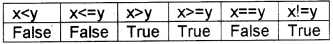4. Logical operators:
Here AND(&&), OR(||) are binary operators and NOT(!) is a unary operator. It is used to combine relational operations and it gives either true(1) or false(O). If x=True and y=False then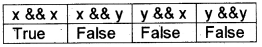Both operands must be true to get a true value in the case of AND(&&) operation If x = True and y = False thenEither one of the operands must be true to get a true value in the case of OR(||) operation If x = True and y = False then5. Conditional operator:
It is a ternary operator hence it needs three operands. The operator is ?:
Syntax: expression ? value if true : value if false. First evaluates the expression if it is true the second part will be executed otherwise the third part will be executed.
eg: If x = 10 and y = 3 then x>y ? cout<<x : cout<<y;. Here the output is 10

6. sizeof():
This operator is used to find the size used by each data type.
eg. sizeof(int) gives 2.

7. Increment and decrement operator:
These are unary operators.
(a) Increment operator (++): It is used to incre¬ment the value of a variable by one i.e., x++ is equivalent to x = x + 1;
(b) Decrement operator (–): It is used to decre¬ment the value of a variable by one i.e., x-is equivalent to x = x – 1.

8. Assignment operator (=):
lt is used to assign the value of a right side to the left side variable.
eg: x = 5; Here the value 5 is assigned to the variable x.